# Balanced Forces

(diff) ← Older revision | Latest revision (diff) | Newer revision → (diff)

## Key Stage 3

### Meaning

Balanced Forces are forces that are the same strength but acting in opposite directions.

### About Balanced Forces

When forces are balanced an object will travel at a constant speed and in the same direction.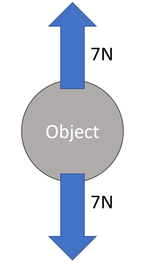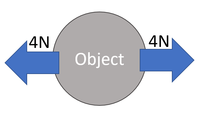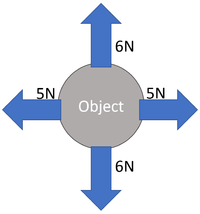The 7N up is balanced by the 7N down. The 4N left is balanced by the 4N right. The 6N up is balanced by the 6N down and the 5N left is balanced by the 5N right.

## Key Stage 4

### Meaning

Balanced forces are force vectors which add together to make 0N resultant force.

### About Balanced Forces

When forces on an object are balanced there is no acceleration (change speed or direction).
Balanced forces are related to Newton's First Law of motion:
An object in motion will tend to stay in motion in a straight line and an object at rest will tend to stay at rest unless an unbalanced force acts upon it.
If the resultant force on an object is zero, then the forces are balanced.

### Examples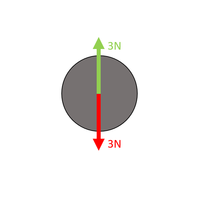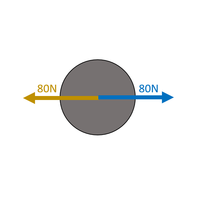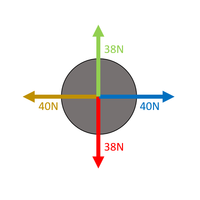$$F_R = F_{up} - F_{down}$$ $$F_R = 3 - 3$$ $$F_R = 0N$$ The forces are balanced. $$F_R = F_{right} - F_{left}$$ $$F_R = 80 - 80$$ $$F_R = 0N$$ The forces are balanced. $$F_{Horiztonal} = F_{right} - F_{left}$$ $$F_{Horiztonal} = 40 - 40$$ $$F_{Horiztonal} = 0N$$ $$F_{Vertical} = F_{up} - F_{down}$$ $$F_{Vertical} = 38 - 38$$ $$F_{Vertical} = 0N$$ The forces are balanced.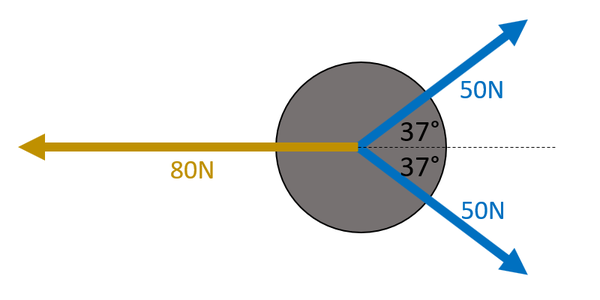Whether these forces are balanced can be found by resolving the blue force vectors into the horizontal. See Resolving Forces. $$F_{right} = 50\cos37 + 50\cos37$$ $$F_{right} = 79.86N$$ Since the angle in the question is given to two significant figures the answer should also be quoted to two significant figures. $$F_{right} \approx 80N$$ $$F_R = F_{right} - F_{left}$$ $$F_R = 80 - 80$$ $$F_R = 0N$$